A mass of 100g strikes the wall with speed 5m/s at an angle as shown in figure and it rebounds with the same speed. If the contact time is $2×{10}^{-3}\text{\hspace{0.17em}}\mathrm{sec}$, what is the force applied on the mass by the wall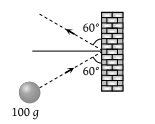(1) $250\sqrt{3}$N to right

(2) 250 N to right

(3) $250\sqrt{3}$N to left

(4) 250 N to left

Concept Questions :-

Application of laws
High Yielding Test Series + Question Bank - NEET 2020

Difficulty Level:

A lead ball strikes a wall and falls down, a tennis ball having the same mass and velocity strikes the wall and bounces back. Check the correct statement

(1) The momentum of the lead ball is greater than that of the tennis ball

(2) The lead ball suffers a greater change in momentum compared with the tennis ball

(3) The tennis ball suffers a greater change in momentum as compared with the lead ball

(4) Both suffer an equal change in momentum

Concept Questions :-

Application of laws
High Yielding Test Series + Question Bank - NEET 2020

Difficulty Level:

A ball of weight 0.1 kg coming with speed 30 m/s strikes with a bat and returns in opposite direction with speed 40 m/s, then the impulse is (Taking final velocity as positive)

(1) $-0.1×\left(40\right)-0.1×\left(30\right)$

(2) $0.1×\left(40\right)-0.1×\left(-30\right)$

(3) $0.1×\left(40\right)+0.1×\left(-30\right)$

(4) $0.1×\left(40\right)-0.1×\left(20\right)$

Concept Questions :-

Application of laws
High Yielding Test Series + Question Bank - NEET 2020

Difficulty Level:

A ball of mass m falls vertically to the ground from a height h1 and rebound to a height h2. The change in momentum of the ball on striking the ground is

(1) $mg\left({h}_{1}-{h}_{2}\right)$

(2) $m\left(\sqrt{2g{h}_{1}}+\sqrt{2g{h}_{2}}\right)$

(3) $m\sqrt{2g\left({h}_{1}+{h}_{2}\right)}$

(4) $m\sqrt{2g}\left({h}_{1}+{h}_{2}\right)$

Concept Questions :-

Application of laws
High Yielding Test Series + Question Bank - NEET 2020

Difficulty Level:

A force-time graph for a linear motion is shown in figure where the segments are circular. The linear momentum gained between zero and 8 second is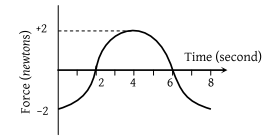1. $-2\pi \text{\hspace{0.17em}}newton×second$

2. $Zero\text{\hspace{0.17em}}newton×second$

3. $+4\pi \text{\hspace{0.17em}}newton×second$

4. $-6\pi \text{\hspace{0.17em}}newton×second$

Concept Questions :-

Newton laws
High Yielding Test Series + Question Bank - NEET 2020

Difficulty Level: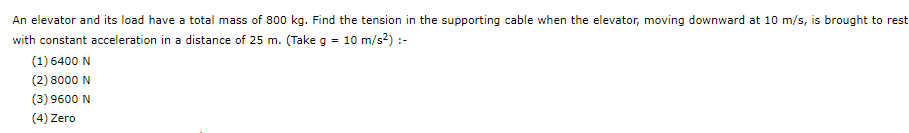Concept Questions :-

Application of laws

Difficulty Level:

Two blocks A and B of masses 3m and m respectively are connected by a massless and inextenisible string. The whole system is suspended by a massless spring as shown in figure. The magnitudes of acceleration of A and B  immediately after the string is cut, are respectively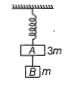1. $g,\frac{g}{3}$

2. $\frac{g}{3},g$

3. $g,g$

4. $\frac{g}{3},\frac{g}{3}$

Concept Questions :-

Spring force
High Yielding Test Series + Question Bank - NEET 2020

Difficulty Level:

One end of the string of length l is connected to a particle of mass m and the other end is connected to a small peg on a smooth horizontal table. If the particle moves in circle with speed v, the net force on the particle (directed towards centre) will be (T represents the tension in the string)

1. T

2. $T+\frac{m{v}^{2}}{l}$

3. T-$\frac{m{v}^{2}}{l}$

4. Zero

Concept Questions :-

Uniform circular motion
High Yielding Test Series + Question Bank - NEET 2020

Difficulty Level:

A spring of force constant k is cut into lengths of ratio 1:2:3. They are connected in series and the new force constant is ${k}^{\text{'}}$. If they are connected in parallel and force constant is  is

(a) 1:6

(b) 1:9

(c) 1:11

(d) 6:11

Concept Questions :-

Spring force
High Yielding Test Series + Question Bank - NEET 2020

Difficulty Level:

In the given figure, represents the total acceleration of a particle moving in the clock wise direction in a circle of radius R=2.5 m at a given instant of time. The speed of the particle is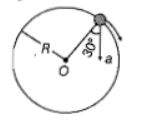(a) 4.5 m/s

(b) 5.0 m/s

(c) 5.7 m/s

(d) 6.2 m/s

Concept Questions :-

Uniform circular motion